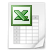### 3x3 in 5x5 & concentric magic square

See on the previous webpage the 3x3 in 5x5 (inlay) magic square, which you can see as a concentric magic square, but you don't find the middle 9 numbers in the 3x3 magic square.

In a 'real' concentric 5x5 magic square the middle 9 numbers of the 5x5 magic square are in the 3x3 inlay magic square. In the border are the 8 lowest and the 8 highest numbers in opposite positions.

Number + 8       =     create border     =   3x3 in 5x5 magic square

 22 18 3 2 20 2 9 4 10 17 12 7 10 17 12 19 7 5 3 15 13 11 5 15 13 11 21 6 1 8 14 9 16 25 14 9 16 1 6 8 23 24 4

Harry White (see website http://budshaw.ca/BorderedMagicSquares.html) teaches us how to create the border.

The border consists of 4 sides with 5 numbers. N.B.: The 4 numbers in the corners are part of two sides. The lowest number is opposite to the highest number, the second lowest number is opposite to the second highest number, and so on  (1+25 = 2+24 = 3+23 = 4+22 = 5+21 = 6+20 = 7+19 = 8+18). You need to puzzle only two of the four sides. In the border are the 8 lowest and the 8 highest numbers. We translate the 8 lowest into 8 sequencial positive numbers. The first number is [the number of the cells of the inlay square +1]/2, so [3x3+1]/2 = 5. So we translate the numbers 8 up to 1 into 5 up to 12 and we translate the numbers 18 up to 25 into -5 up to -12; see table below.

 12 1 11 2 10 3 9 4 8 5 7 6 6 7 5 8 -5 18 -6 19 -7 20 -8 21 -9 22 -10 23 -11 24 -12 25

Each side consists of 2 + 3 numbers, 2 positive & 3 negative or 2 negative & 3 positive numbers. The sum of the numbers of each side must be 0. Because of the corners you need two numbers double. You must create two sequences with 2 numbers and two sequences with 3 numbers. The two double numbers are each in one sequence, both in one sequence and none in one sequence; see table below:

 5 6 7 8 9 10 11 12 84 10 11 21 9 12 21 5 7 9 21 6 7 8 21

N.B.: 5+6+7+8+9+10+11+12+7+9 = 84 and the sum of each sequence must be 84/4 is 21.

Fill in one sequence with positive numbers and one sequence with negative numbers in two sides and put the double numbers 7 and 9 in the corners. Fill in the other two sides.

 -9 -5 10 11 -7 -9 -5 10 11 -7 -6 6 -6 -8 8 -8 12 -12 12 9 7 5 -10 -11 9

Use the above mentioned table to translate the numbers and fill in the 3x3 inlay.

 22 18 3 2 20 22 18 3 2 20 7 19 7 10 17 12 19 5 21 5 15 13 11 21 25 1 25 14 9 16 1 6 8 23 24 4 6 8 23 24 4

You can use this method to construct magic squares of odd order from 5x5 to infinite. See on this website

See how the concentric magic square on this website is growing bigger and bigger:5x5, 3x3 in 5x5 & concentric.xls